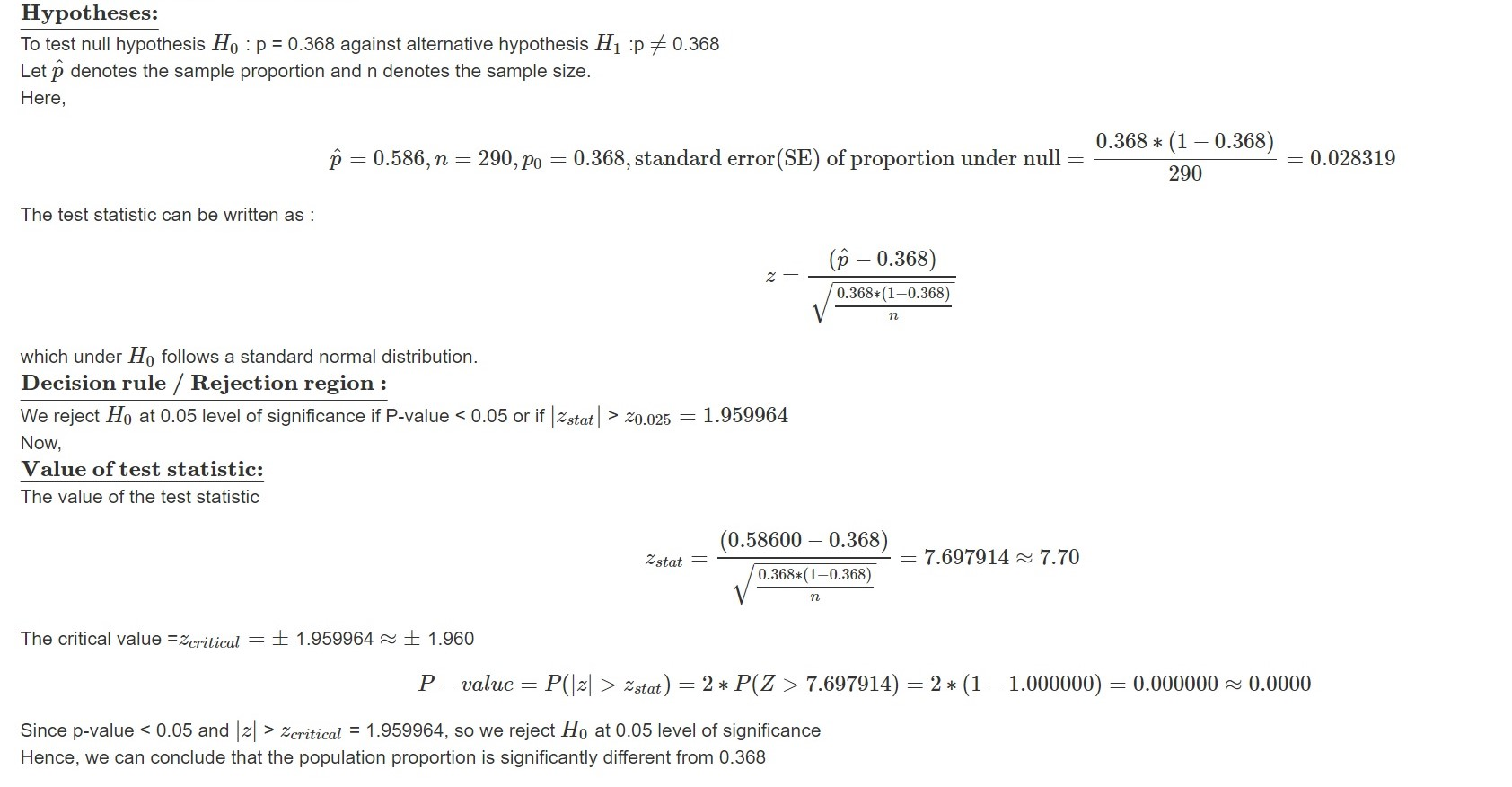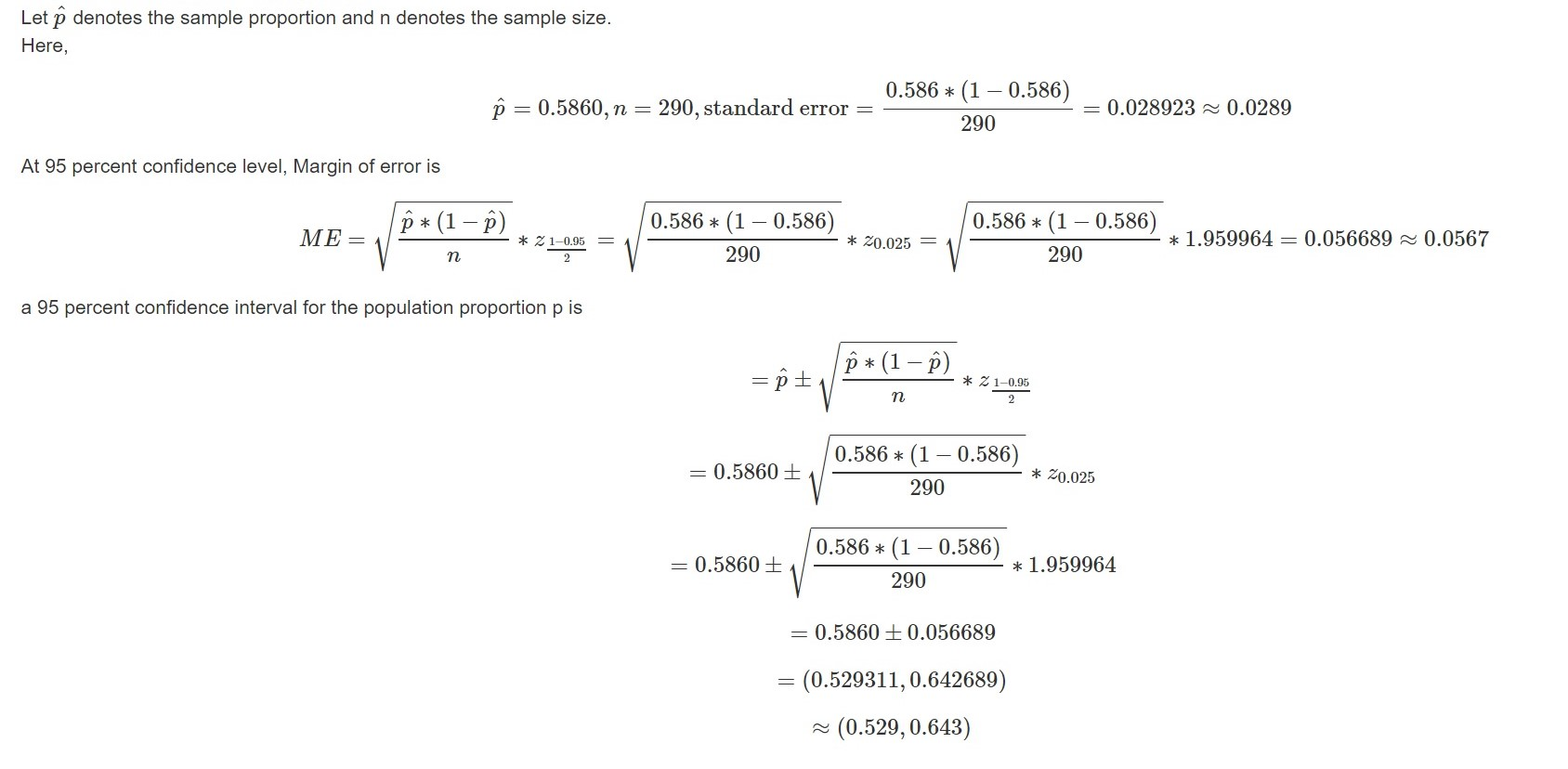##### Question

In: Statistics and Probability

# A random sample of 124 women over the age of 15 found that 3.68% of them...

A random sample of 124 women over the age of 15 found that 3.68% of them have been divorced. A random sample of 290 men over the age of 15 found that 5.86% have been divorced. Assuming normality and using a 95% significance level, test the claim that the proportion of divorced women is different than the proportion of divorced men.

For this scenario, provide a hypothesis test with all six steps and provide both the critical value and the p-value.

provide a confidence interval estimate of the true difference in proportions between men and women who have been divorced.

Explain how your hypothesis test in 12 and your confidence interval in 13 are consistent.

## Solutions

##### Expert Solution

Let p denotes the true proportion of divorced men over the age 15.

i)ii)Since the confidence interval does not includes 0.368, so at 95% level of confidence or at 5% level of significance, we can conclude that there is not sufficient evidence to support the claim that the proportion of divorced women is different than the proportion of divorced men.

## Related Solutions

##### A random sample of 500 students were selected and it was found that 345 of them...
A random sample of 500 students were selected and it was found that 345 of them were satisfied with their field. Construct a 95% confidence interval for the true proportion of students who are satisfied with their field. (i)Identify n, p̂, q̂, and ??/2. ? = p̂ = q̂ = ??/2 = (ii)Calculate the margin of error, E. (iii)Construct the 95% confidence interval
##### A simple random sample of 10 people shopping at Target found a sample mean age of...
A simple random sample of 10 people shopping at Target found a sample mean age of 27 and a sample standard deviation of 4.27 yrs. Can the market research team definitively declare that the mean age of the population of shoppers at Target is less than 30? Suppose that the level of significance is 0.05.
##### A random sample of 150 visitors traveling in Hawaii found that 14% of them hiked the...
A random sample of 150 visitors traveling in Hawaii found that 14% of them hiked the Legendary Na Pali Coast. Create a 94% confidence interval for the population proportion of visitors hiking the Na Pali Coast. Enter the lower and upper bounds for the interval in the following boxes, respectively. You may answer using decimals rounded to four places or a percentage rounded to two. Make sure to use a percent sign if you answer using a percentage.
##### A random sample of 150 WCC students found that 68 of them were Latino/a. a) At...
A random sample of 150 WCC students found that 68 of them were Latino/a. a) At the 5% level of significance can we prove that over 40% of all WCC students are Latino/a? State and test appropriate hypotheses. State conclusions. b) Find the p-value of the test in (a). c) If an error was made in (a), what type was it?
##### 15. In a random sample of 60 adults, 55% of them reported having a fear of...
15. In a random sample of 60 adults, 55% of them reported having a fear of public speaking. (1)(1.5 points) Are the assumptions for constructing a confidence interval for the population proportion satisfied? Explain. (2)(5 points) Construct a 99% confidence interval for the population proportion of adults who have a fear of public speaking. (3)(2.5 points) Interpret the 99% confident interval. (4)(3.5 points) Estimate the sample size needed so that a 99% confidence interval will have a margin of error...
##### In a simple random sample of 1600 people age 20 and over in a certain​ country,...
In a simple random sample of 1600 people age 20 and over in a certain​ country, the proportion with a certain disease was found to be 0.110 ​(or 11.0​%). Complete parts​ (a) through​ (d) below. a. What is the standard error of the estimate of the proportion of all people in the country age 20 and over with the​ disease? SE est=Answer______ (Round to four decimal places as​ needed.) b. Find the margin of​ error, using a​ 95% confidence​ level,...
##### Is there a relation between murder and arson? A random sample of 15 Midwest cities (over...
Is there a relation between murder and arson? A random sample of 15 Midwest cities (over 10,000 population) gave the following information about annual number of murder and arson cases. (Reference: Federal Bureau of Investigation, U.S. Department of Justice.) City 1 2 3 4 5 6 7 8 9 10 11 12 13 14 15 Murder 12 18 23 15 21 20 8 22 17 16 9 7 11 13 19 Arson 37 19 157 29 165 38 7 105...
##### A random sample of 150 companies found that 75 of them use social-media tools and technologies....
A random sample of 150 companies found that 75 of them use social-media tools and technologies. Determine the LOWER confidence limit (LCL) for an 80% confidence interval for the population proportion.
##### 1. Over 9 months, a random sample of 100 women were asked to record their average...
1. Over 9 months, a random sample of 100 women were asked to record their average menstrual cycle length (in days). The sample average was 28.86 days, with a sample standard deviation of 4.24 days. (a) Calculate the lower bound for the 90% confidence interval for the true average menstrual cycle length. (b) Calculate the upper bound for the 90% confidence interval for the true average menstrual cycle length. (c) Interpret the confidence interval found in (a,b) in terms of...
##### The age of distribution of the Canadian population and the age distribution of a random sample...
The age of distribution of the Canadian population and the age distribution of a random sample of 455 residents in the Indian community of Red Lake Village (Northwest Territories) are shown in the chart. Use a 5% level of significance to test the claim that the age distribution of the general Canadian population fits the age distribution of the residents of Red Lake Village. Age (years) Percent of Canadian Population Observed Number in Red Lake Village under 5 7.2% 47...